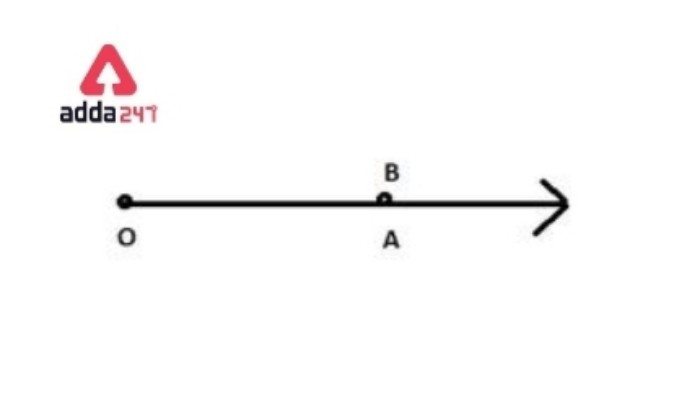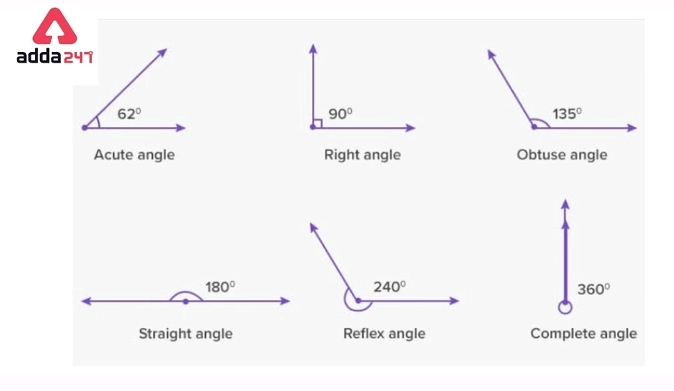Online Tution   »   Important Question   »   Types of Angles

# Types of Angles in Maths with Definition, Degrees & Examples

## Types of Angles in Geometry with Definition

In geometry, There are various types of angles. One of the most important and primary units is Angle. An angle is formed by when two rays or lines are joined at their common endpoint. Not only in maths we use angles in our daily life aspects, starting from a pizza slice to construct buildings, dams, and statues. Angle is an integral part of Civil Engineering. In this article, we will learn about different types of angles and their properties in different aspects.

## Types of Angles: What is an angle?

Angles are the fundamental factors in geometry as well as daily life activities.
Basically, when two lines intersect at a common point, the measurement of the opening between two lines is known as the angle. Angle is represented by the symbol ∠.
Units used in the measurement of an angle are degree and radian.

## Types of Angles: Parts of an angle

From an angle, There are mainly 2 parts  –
• Vertex-  In an angle, The common meeting point of the two lines is known as Vertex.
• Arms– Two lines or rays which intersect at a common point, are called arms. Two arms are -the terminal side and the initial side.

## Types of angles in Maths with Degrees

We can classify angles based on two aspects –

•According to measurement

• According to the direction of rotation

According to measurement –
based on angle measurement we can classify into 7 classes. Each angle has different identification and shape.
Let’s go through those 6 types and their properties.

1. Zero angle

Zero angles are formed when two rays of the angles are pointed in the same direction and rays start from a common point that measures exactly 0°.Zero angle2. Acute Angle
An Angle that lies between greater than  0° and less than 90°, is known as an Acute angle.
0°<Acute angle >90°

3. Right Angle
The right angle looks like the English alphabet letter ‘L’  and can be easily observed. This angle is 90°.
Right angle =90°

4. Obtuse Angle
The angle in which the measurement is less than 180° but greater than 90° it is called an obtuse angle.

90°<Obtuse Angle  >180°

5. Straight Angle
When intersecting two rays have formed an angle that measures exactly 180° is known as a Straight angle. It looks like a straight line that’s called so.
Straight angle – 180°

6. Reflex Angle
A reflex angle lies between greater than 180° and less than 360°.

180° < Reflex Angle >360°

7. Complete Angle
When an angle is exactly equal to 360°, this is called a complete angle.

## Types of angles  Table for Class 5 and 9

Here we provide a summary of the types of angles-

 Types of angles Angle measurement Zero angle Equals 0° Acute angle Greater than 0°, less than 90° Right angle Equals exactly 90° Obtuse angle Greater than 90°, less than 180° Straight angle Equals 180° Reflex angle Greater than 180°, less than 360° Complete angle Equals 360°● According to the direction of rotation –
We can classify angles on the basis of the direction of rotation in two categories-
Positive angles:  If the measurement of the angles is to be done in the anti-clockwise direction starting from the base of the angle.
•  Negative angles: Angles that are measured in the clockwise direction starting from the base of the angle.

## Pairs of angles

Pairs of angles are described as When two angles appear in groups of two to display a certain geometrical property. There is some special relationship between those pairs of angles.
Some types of angle pairs are-  complementary angles, supplementary angles, adjacent angles, vertical angles, and corresponding angles.

## Types of pairs of angle

Some types of pairs of angles are –

Complementary angles

When two angles are called complementary angles, it means the sum of their measures is 90°. Those types of angles complement each -other.

Supplementary angles
When the sum of two angles measures 180°.  Those angles are the supplement of
the each other, is known as supplementary angles.

When two angles share a common vertex and side, They are called Adjacent angles.
Non-adjacent angles are formed if two angles have no common vertex or side.

vertical angles
When two lines or rays intersect in a common point and results four angles are formed. In those four angles, we observed that vertically present two opposite angles are equal, these pair of angles is called verticle angles.

corresponding angles
Corresponding angles are a pair of angles that forms when two parallel lines are intersected by a transversal.

## Types of angles in parallel lines

Parallel lines mean a pair of lines that never intersect and never meet with each other in a plane. When a transversal intersects a pair of parallel lines different types of pairs of angles are formed. Some of the pairs of angles are listed below-
•  Corresponding angles
•Alternate interior angles
• Alternate exterior angles
• Vertically opposite angles
•  Linear angles

## Types of angles exercises

Q. An angle that is greater than 180°, less than 360° is known as __
a) Right angle. b) Obtuse angle c) straight angle d) Reflex angle.
Because the Reflex angle lies between greater than 180° and less than 360°.

Q.In a pair of  Supplementary angles one of the angle measures is 45°. What is the measurement of the other supplement angle?
a) 45° b) 130° ( c) 135 ° (d) None of those
Answer- we know that In a pair of supplementary angles, the sum of two angles is 180°.
In given supplementary angles,  one angle is 45°.
Then the other angle is (180°-45°) = 135°

Option ( c) is correct.

Q.In a pair of complementary angles one of the angle measures is 30 °. What is the measurement of the other complement angle?
a) 150° b) 60° ( c) 90 ° (d) None of those

Answer- In Complementary angles, it means the sum of their measures is 90°.

In given complementary angles,  one angle is 30°.
Then the other angle is -(90°-30°) = 60°

Hence option (b) is correct.

Q.An Angle  P measures 150° in protractor. In  Which category of angle does Angle P fall?

a) Right angle. b) Obtuse angle c) straight angle d) Reflex angle.

Answer: Angle P fall between 90° and 180°.We know that angle that lies between less than 90° and greater than 90° is called an obtuse angle.
Therefore angle P is an obtuse angle.

Q. In a protractor, an Angle  X measures 20°. Which type of angle does Angle X belong to?

a) Right angle. b) Obtuse angle c) Acute angle d) Reflex angle.

Answer- Angle X lies between 0° and 90°.
An Angle  Which angle measures greater than  0° but less than 90°, is known as an Acute angle.
0°<Acute angle >90°
Therefore, angle X is an Acute angle.

Q. An angle that is greater than 90°, less than 180° is known as __
a) Right angle. b) Obtuse angle c) straight angle d) Reflex angle.

An obtuse angle lies between less than 180° and greater than 90°.

## Types of angles Properties

1. An Angle  Z measures 183 ° in the protractor. In  Which category of angle does Angle Z fall?
2. In a pair of complementary angles, one of the angle measures is 80°. What is the measurement of the other complement angle?
3. An angle that is greater than 0°, less than 90° is known as __
4. An Angle  W measures 75 ° in the protractor. In  Which category of angle does Angle W fall?

Related Post:

## Types of Angles in Maths with Definition, Degrees & Examples- FAQs

Q.What are the 7 types of Angles?

A- Based on angle measurement we can classify into 7 types –
Zero Angel, Acute Angle, Obtuse Angle, Right Angle, Straight Angle, Reflex Angle, Complete Angle.

Q.How an angle is  Measured?

A-For measurement of an angle use protractor. a simple protractor looks like a semicircular disk graduated in degrees from 0° to 180°.

Q.What is a Reflex Angle?

A-A reflex angle is an angle that lies between greater than 180° and less than 360°.

Q.What are the types of triangles based on the direction of rotation?

A-We can classify angles on the basis of the direction of rotation in two categories- Positive angles ( measurement done   in the clockwise direction) and Negative angles (measurement done   in the clockwise direction)

Q.What is the measurement of the right angle?

A-The right angle exactly measures 90°. The right angle looks like the English alphabet letter ‘L’  and can be easily observed.

Q.What is a Zero Angle?

A-Zero angles are formed when two rays of the angles are pointed in the same direction and rays start from a common point that measures exactly 0°.

Sharing is caring!

Thank You, Your details have been submitted we will get back to you.